In addition to the examples of vector spaces discussed in the text and elsewhere, we also discussed one example in which the operations of vector addition and scalar multiplication wouldn't normally be called addition'' or multiplication''.

• Our vectors, which we denote as usual by greek letters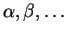, are the positive real numbers,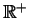.
• Our field of scalars (denoted by roman letters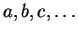) can be either the reals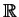or the rationals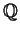. We'll writebut we get a vector space withas well.

• To add'' two vectors, we multiply the corresponding real numbers. That is, the vector sum of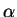and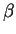is the product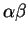.
• The scalar product of a scalar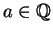and the vector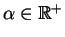, we compute the power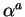.

Let's check that this satisfies the necessary properties:

Ifandare positive real numbers, so is their product.
Multiplication of real numbers is associative, so no problem.
Multiplication of real numbers is commutative.
The positive real number 1 acts as the identity element for vector addition, since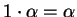.
For any vector, there is another vector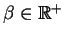so when the two vectors are added'', the result is the identity element above. In this case, the inverse ofis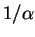, since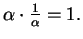closure of scalar multiplication
For any scalarand any vector, the scalar multipleis still in.
neutrality of 1
When we compute the scalar multiple of the multiplicative identity in our field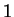with any vector, we should get the original vector. That works fine: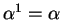vector distributive law
Multiplying a scalar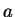times the sum of two vectorsand: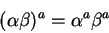scalar distributive law
The sum of two scalars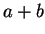times a vector:So we see that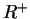is a vector space over, with an appropriate interpretation of vector addition and scalar multiplication.

Note also that in this case, a linear combination'' works out to be very like factoring. For example, we can express the vector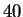as a linear combination of the vectors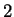and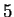by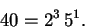Note that there may be more than one way to express the same vector as a linear combination of two others. For example, if our underlying field is, then there are scalars inequal to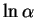and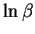, and so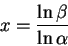is also a scalar. But then if we have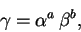we also have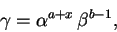since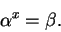More concretely, since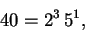we also have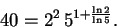So we can expressas a linear combination ofandin many different ways. What is the dimension ofas a vector space over? Can you give a proof?

The situation is more complicated if we consideras a vector space over, since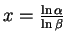will be rational for someand, and not for others. What do you think the dimension of this vector space is?

Scott Sutherland February 10, 2005